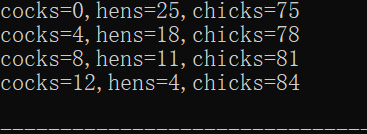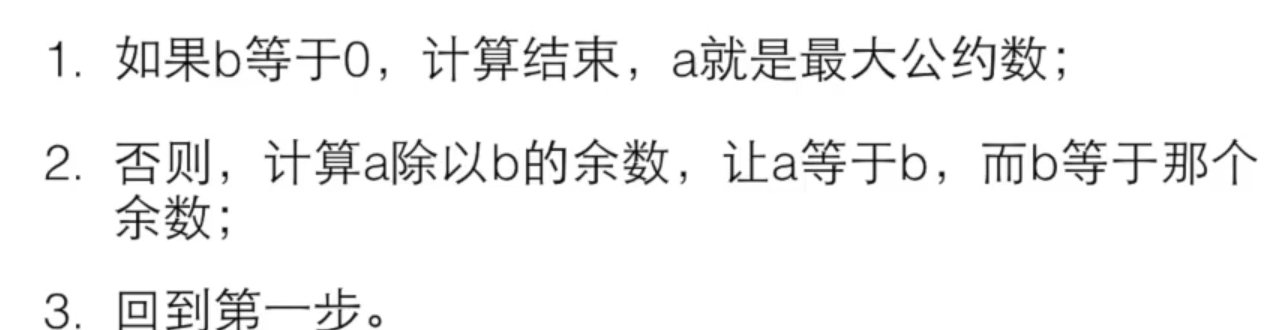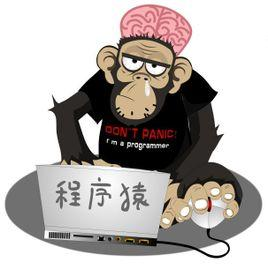WHCSRL 技术网

# 初学者之循环_m0

## 一：穷举法

### 例题：我国古代数学家张丘建在《算经》一书中曾提出过著名的“百钱买百鸡”问题，该问题叙述如下：鸡翁一，值钱五；鸡母一，值钱三；鸡雏三，值钱一；百钱买百鸡，则翁、母、雏各几何？（

意思是公鸡一个五块钱，母鸡一个三块钱，小鸡三个一块钱，现在要用一百块钱买一百只鸡，问公鸡、母鸡、小鸡各多少只）

``​#include <stdio.h>int main(){		return 0;} ​``

首先可以确定的是，公鸡最多22只，母鸡33只，小鸡仔叁佰只；

``​	int cocks,hens,chicks;	for(cocks=0;cocks<=20;cocks++){		for(hens=0;hens<=33;hens++){			for(chicks=0;chicks<=300;chicks++){							}		}	} ​``

``````if(5*cocks+3*hens+chicks/3.0==100)			printf("cocks=%%d,hens=%%d,chicks=%%d
",cocks,hens,chicks);``````对于结果较多的穷举法，我们以可以在其中计入计数器：

``````#include <stdio.h>int main(){	int one,two,five;	int x;	printf("请输入x元");	scanf("%%d",&x);	int cnt=0;	for(one=0;one<=100;one++){		for(two=0;two<=50;two++){			for(five=0;five<=20;five++){				if(one*1+two*2+five*5==100*x){					printf("%%d个一分%%d个两分和%%d个五分组成%%d元
",one,two,five,x);					cnt+=1;				}			}		}	}	printf("%%d",cnt);	return 0;	}``````

## 二：辗转相除法求最大公约数

（辗转相除法求最大公约数大家可以借鉴数学，不在c语言范畴内了^-^）``​#include <stdio.h>int main(){	int a,b;	scanf("%%d%%d",&a,&b);	int c=0;	while(b!=0){		c=a%%b;		a=b;		b=c;	}	printf("%%d",a); } ​``

（从我看的网课截下来的）